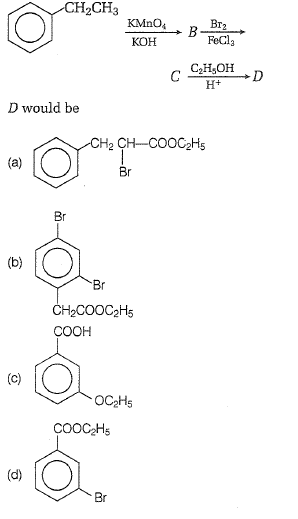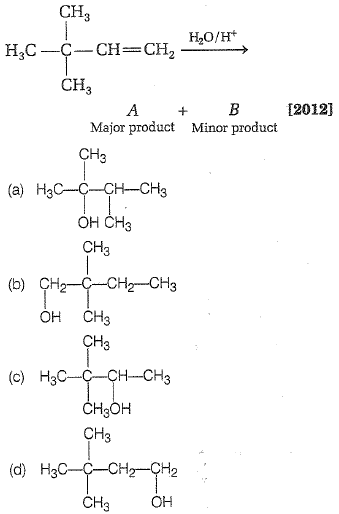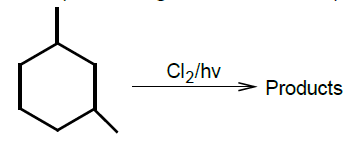In a set of reactions, ethyl benzene yielded a product D.Concept Questions :-

Aromatic Hydrocarbons - Benzene - Structure, Preparation & Chemical Reactions
High Yielding Test Series + Question Bank - NEET 2020

Difficulty Level:

Nitrobenzene on reaction with conc. HNO3/H2SO4 at 80-100$°$C forms which one of the following products?                                                                                            

(a) 1,2-dinitrobenzene

(b) 1,3-dinitrobenzene

(c) 1,4-dinitrobenzene

(d) 1,2,4-trinitrobenzene

High Yielding Test Series + Question Bank - NEET 2020

Difficulty Level:

Propene, CH3-CH=CH2 can be converted into 1-propanol by oxidation. Indicate which set of reagents amongst the following is ideal to effect the above conversion?

1. KMnO4(alkaline)

2. Osmium tetroxide (OsO4/CH2Cl2)

3. B2H6 and alk H2O2

4. O3/Zn

High Yielding Test Series + Question Bank - NEET 2020

Difficulty Level:

In the following reaction,Concept Questions :-

Alkanes, Alkenes and Alkynes - Chemical Properties
High Yielding Test Series + Question Bank - NEET 2020

Difficulty Level:

CH3-C$\equiv$C-H$\stackrel{{\mathrm{CH}}_{3}\mathrm{MgBr}}{\to }$CH4 + (A)(B); (B) will be

1. H3C-CH2-CH2-COOH                                2. CH3-C$\equiv$C-CH3

3. H3C-C$\equiv$C-COOH                                     4. H3C-CH=CH-COOH

Concept Questions :-

Alkanes, Alkenes and Alkynes - Chemical Properties
High Yielding Test Series + Question Bank - NEET 2020

Difficulty Level:

The monochlorinated products (excluding stereo-isomers) obtained from the reaction is:(1) 4            (2) 5              (3) 6            (4) 7

Concept Questions :-

Alkanes, Alkenes and Alkynes - Chemical Properties
High Yielding Test Series + Question Bank - NEET 2020

Difficulty Level:

3-hexyne can be converted to trans-3-hexene by the action of:

1. ${\mathrm{H}}_{2}$ - Pd/ ${\mathrm{BaSO}}_{4}$                       2. Li-Liq. ${\mathrm{NH}}_{3}$

3. ${\mathrm{H}}_{2}$ - Pt ${\mathrm{O}}_{2}$                                4. ${\mathrm{NaBH}}_{4}$

Concept Questions :-

Alkanes, Alkenes and Alkynes -Methods of Preparation
High Yielding Test Series + Question Bank - NEET 2020

Difficulty Level:

Which one of the following has the shortest carbon-carbon bond length?

1. Benzene                                  2. Ethene

3. Ethyne                                    4. Ethane

Concept Questions :-

Aliphatic Hydrocarbon- physical properties
High Yielding Test Series + Question Bank - NEET 2020

Difficulty Level:

Reaction of HBr with propene in the presence of peroxide gives

1. iso-propyl bromide

2. 3-bromo propane

3. allyl bromide

4. n-propyl bromide

High Yielding Test Series + Question Bank - NEET 2020

Difficulty Level:

In Friedel-Craft's synthesis of toluene, the reactants in addition to anhydrous ${\mathrm{AlCl}}_{3}$ are

(a)

(b) ${\mathrm{C}}_{6}{\mathrm{H}}_{5}\mathrm{Cl}$ + ${\mathrm{CH}}_{3}\mathrm{Cl}$

(c) ${\mathrm{C}}_{6}{\mathrm{H}}_{6}$ + ${\mathrm{CH}}_{4}$

(d) ${\mathrm{C}}_{6}{\mathrm{H}}_{6}$ + ${\mathrm{CH}}_{3}\mathrm{Cl}$

Concept Questions :-

Aromatic Hydrocarbons - Benzene - Structure, Preparation & Chemical Reactions